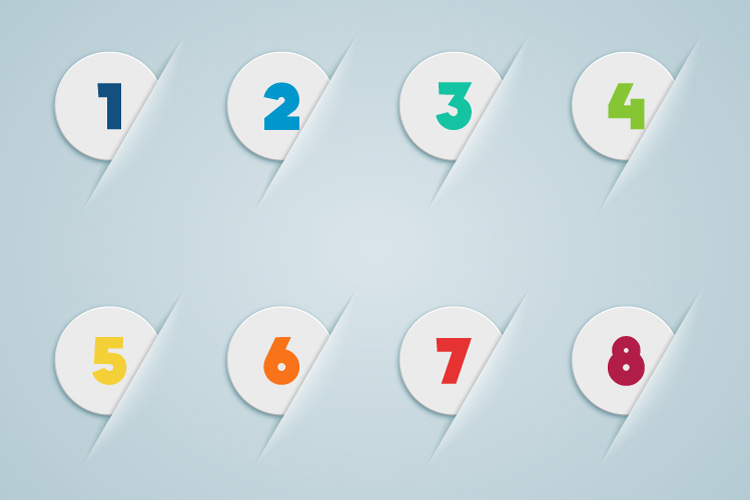# Nabila is smart! Nafiza smarter!!

1,023.7K Views

Nafiza is a very funny girl!
She plays with numbers.
All her numbers are rational.
And she writes the numbers by p/q style.
Here both p and q are digits!!
Now her friend Nabila turns all the numbers written in p/q style into points.
Nabila writes them in the following way:
p/q=0.ABCDEF
Here it’s ensured that before points there must be a zero. A,B,C,D,E and F are zero/nonzero digits!!
Now Nabila defines a function MULTmin , and declares MULTmin=A*B*C*D*E*F
And she finds all the MULTmin value of Nafiza’s numbers.
Then she tells one of her MULTmin value.
Hearing the value Nafiza understands what is the rational number chosen by Nabila.
HOW ? Find the way!she have already calculated all of them before and they were distinct .obviously it was about her numbers whom she play with so she would have known them.“And she writes the numbers by p/q style.”
“Now her friend Nabila turns all the numbers written in p/q style into points.”
So it’s Nabila who only knows the values of MULTmin.
And Nafiza doesn’t know because she never turns them into points!!

on 4th June 2016.she would have calculated through calculator  there must be something strange so she would remember it.it is easy to write  in rational form as it is small so it always help

on 5th June 2016.

To write a fraction in the form p/q= 0.abcde. that is to ensure there is a zero before decimal q must be greater than p that is q>p.Now it we choose highest digit for q that is 9 then any number written in the form p/q or p/9 where p=1,2,…9 when converted to fraction attain the form p/9=0.ppppppp…

that is 1/9=0.11111
2/9=0.2222
3/9=0.3333…

now after hearing the MultMIn value Nabila only need to find its fifth root to find the number.And to find the fifth root she only need to tell the last digit of the MultMin value .Because for every digit its fifth square ends in the same digit[assuming p and q are single digit numbers  ]

q can be 1 to 9
if q is 2,4,5,8, mult mn is zero as in those cases some of A,B,C,D,E,F, WILL BE ZERO.
IF Q IS 7 P IS LESS THEN 7 AND ANY NUMBER MULTIPLIED BY  142857 (1/7=0.142857) LESS THEN 7 HAVE SAME DIGITS.SO MULTI MIN FOR ALL 1/7 TO 6/7 WILL BE SAME (CYCLIC NUMBER).IF Q IS 9 THEN IT IS ALL ABOUT SIXTH ROOT AS 2/9 =0.222222…..
SIMILARLY FOR OTHERS.
IF Q IS 3  THEN IT  CAN BE REPRESENTED IN 9 CASE AS 2/3=6/9     1/3=3/9
IF Q IS 6 THEN 2/6 IS SAME AS 1/3,          4,6 AS 2/3         3/6 AS 1/2 ONLY 5/6 IS .833333
SO THE MULTIMINN NABILA REFEREED WAS 8 *3*3*3*3*3 AS IT IS THE ONLY ONE BY WHICH WE CAN DIRECTLY IDENTIFY THE NUMBER

EDIT
I FORGOT ABOUT 1/6 AND 1/9 IF MULTIMIN IS 1 THEN NUMBER WILL BE 1/9
AND  IF IT IS 6^5 THEN IT IS 1/6 AS IT IS 0.166666
SO SHE WILL  SEE  IF  MULTIMINN IS NOT SIXTH POWER OR FIFTH POWER OF A DIGIT THEN NUMBER WILL BE  5/6
OTHERWISE THOSE UNIQUE CASES ARE PREVIOUSLY MENTIONED